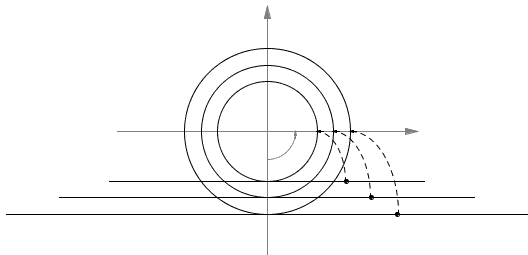Area of a Circle by Rabbi Abraham bar Hiyya Hanasi

RABH is an acronym for Rabbi Abraham bar Hiyya Hanasi - a famous Jewish philosopher and scientist who lived in the 11-12th centuries in Spain and France. In one of his works on mensuration (Chibbur ha-Meshicha weha-Tishboreth), RABH has developed an argument as to why half the product of the circumference $L$ of a circle of radius $R$ by the radius equals its area $A$, $A = \frac{1}{2}LR$. It is said that the book has been written in Hebrew (and not in Arabic as would have been customary at the time) for the benefit of the French Jewry not conversant in Arabic. Probably towards the end of his life the book has been translated into Latin by Plato of Tivoli, under the title Liber Embadorum (1145). The latter was widely used across Europe.

From the partly preserved 1913 edition (Berlin) by Dr. Michael Guttmann, we have the following illustration of the method:The applet below purports to enliven the illustration but, otherwise serves the same purpose. I borrowed the idea for the applet from the site of Boaz Tsaban whose 2001 article brought RABH's chef d'oeuvre to modern audience.

Presently, in Windows only Firefox runs Java applets and that after setting up in Java the site they are coming from as trusted.

 What if applet does not run?

The idea is of course that the disk (i.e., the region inside a circle) is looked at as consisting of layers of circles which when cut, peeled off and flattened form a triangle of area equal to that of the circle. The base of the triangle is the circumference and the altitude the radius of the circle, implying $A = \frac{1}{2}LR$. (In modern terms we establish the formula $A = \frac{1}{2}2\pi R\cdot R = \pi R^2$.)

Tsaban shows how RABH's derivation could be justified using the modern tools of Integral Calculus. I'll give a little different demonstration.

Let there be in the plane two coordinate systems $(x, y)$ and $(u, v)$ and a bijective mapping $g$ from region $A$ in the first onto region $B$ in the second. The areas of the two regions are $|A| = \iint_{A}dA$ and $|B| = \iint_{B}dB$. The relation between $dA$ and $dB$ is simple

$dB = |J|dA,$

where $J$ is the Jacobian of the mapping $g(x, y) = (u(x, y), v(x, y))$:

$J = \begin{vmatrix} \frac{\partial u}{\partial x} & \frac{\partial u}{\partial y} \\ \frac{\partial v}{\partial x} & \frac{\partial v}{\partial y} \end{vmatrix}$

We shall consider a specific mapping from RABH's triangle to the circle illustrated with the diagram below. $(u, v)$ is the Cartesian system corresponding to the polar coordinate $(r, \phi)$ with the $0$ of $\phi$ on the negative $y$-axis and the counterclockwise direction being positive:We then have $r = -y, \phi = \frac{x}{r}$ and so

\begin{align} u & = y\cdot sin\phi = y\cdot sin\frac{x}{y} \\ v & = y\cdot cos\phi = y\cdot cos\frac{x}{y} \end{align}

With these, we compute the Jacobian:

\begin{align} J & = \begin{vmatrix} cos\frac{x}{y} & sin\frac{x}{y} - y\cdot cos\frac{x}{y} \\ -sin\frac{x}{y} & cos\frac{x}{y} + y\cdot sin\frac{x}{y} \end{vmatrix} \\ & = sin^{2}\frac{x}{y} + cos^2\frac{x}{y} = 1, \end{align}

implying $|B| = \iint_{B} dB = \iint_{A}{1} \ dA = |A|$, as promised.

Reference

1. D. Garber and B. Tsaban, A Mechanical Derivation of the Area of the Sphere, Am Math Monthly, 108 (2001), 10-15

π: Applications and Calculations• Cavalieri's Principle
• Derivative of Sine and Cosine
• Distance From a Point to a Straight Line
• Estimating Circumference of a Circle
• Maximum Volume of a Cut Off Box
• Mistrust Intuition of the Infinite
• Naturally Discontinuous Functions
• Rolle's and The Mean Value Theorems
• Function, Derivative and Integral
• Schwarz Lantern
• Two Circles and a Limit
• Deceptive Appearances
• Problem 4010 from Crux Mathematicorum
•